Related Articles

# ToDo App in C Language

• Difficulty Level : Medium
• Last Updated : 08 Jun, 2021

ToDo List App is a kind of app that generally used to maintain our day-to-day tasks or list everything that we have to do, with the most important tasks at the top of the list, and the least important tasks at the bottom. It is helpful in planning our daily schedules. We can add more tasks at any time and delete a task that is completed.

Features:

In this version of the ToDo list, the user will be getting four options:

• See all the tasks or View all the ToDos that were added to the app.
• Delete any ToDo from the list of ToDos.
• Exit from the app.

Approach:

This program involves the basic concepts like variables, data types, structure, string, loop, inserting a node into the linked list at any position, deleting a node from the linked list at any position, linked list traversal, etc. The approach followed for constructing the ToDo application is as follows:

• The splash screen will display the name of the application and the developer: This is done using some statements inside the printf() function (the predefined function used to print the (“character, string, float, integer, octal and hexadecimal values”) and some predefined-functions.
• The second screen will present the user with a list of four options i.e. Add, Delete, View, and Exit: This is achieved using Switch-cases.
• Depending upon the user selects a corresponding function screen will be displayed: Functions for each task are created. Since C Language is a function or procedure based language, so we should make functions for specific jobs.
• All the ToDos will be written inside the data part of the node of the Linked List. The linked list should be declared globally so that data (our ToDos) will not get lost if a function’s execution is over. And by declaring it globally all functions can use the same data that is inside the linked list.

Below are the functionality of the above program:

• The Splash screen: This consists of the name of the application and the developer. The code is written inside a function named interface():
• interface() function contains some printf statements and a predefined function called system().
• The system() function is a part of the C/C++ standard library. It is used to pass the commands that can be executed in the command processor or the terminal of the operating system and finally returns the command after it has been completed.
• system(“Color 4F”) will change the color of the console i.e. background (4) and the text on the console i.e. foreground (F).
• system(“pause”) will pause the screen so the user will get a message: Press any key to continue . . .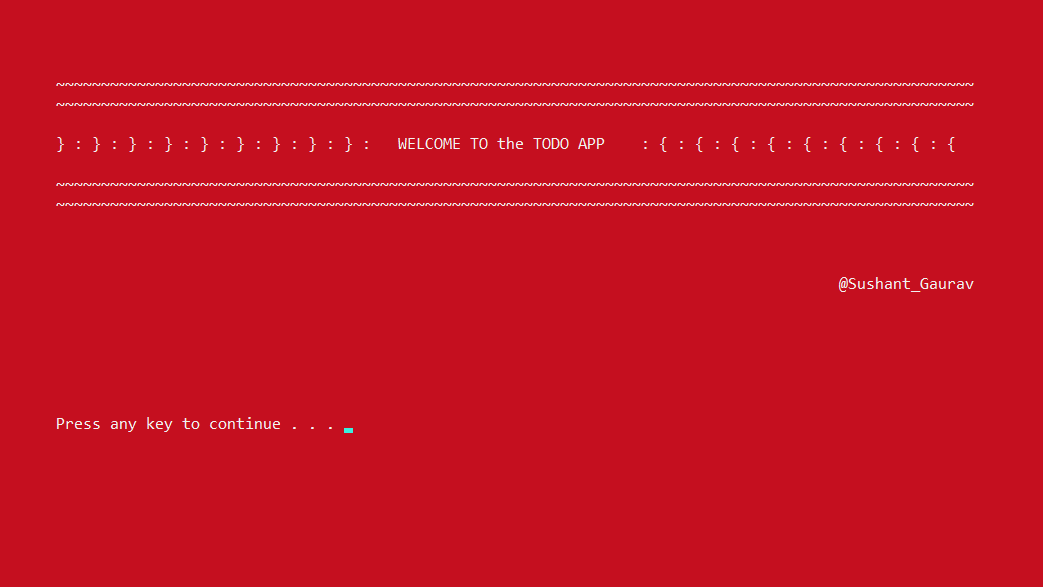• main() function: Use a simple switch case inside an infinite while-loop so that users will get to make choices every time and provide choices with the help of the printf() function and taking user’s input using the scanf() function. According to the input, the specific case will be executed and the required function will be called.
• Linked List: Linked list named Todo is made using the structure concept of C and using the typedef we are renaming it to Todo. This Linked list consists of three parts –
• The data part is made as an array of characters i.e., char buffer. The ToDos can be large so declaring the size of the array as 101.
• The node part contains the address of the next node i.e. *next.
• An integer types variable (int count) that will take account of the number of nodes and will help in the numbering of ToDos in further defined functions.
• As in a singly linked list, a start pointer (In this case- todo *start) is used to get the address of the first node, it is declared and kept NULL inside it (Initially pointing to NULL).
• seetodo() function: Four concepts are coded in this function. These are as follows:
• system(“cls”): to clear the screen or the console. It can be avoided if anyone wants to see all the previous operations or inputs done by the user.
• Creating an object of the structure variable i.e. *temp to access the linked list structure. This temp variable will point to start initially. We can output Empty ToDo if the start is equal to NULL. This means that our list is empty.
• Using a simple linked list traversal concept i.e., print the data part, node by node until the last node we can print all the ToDos. The while loop will execute till the last node, printf() inside it will print the numbering of ToDos, and puts() function will print the data which is in the form of a string of characters. fflush() is a predefined function, its purpose is to clear (or flush) the output buffer and move the buffered data to the console.
• Finally using the system(“pause”) to pause the screen until the user presses any key.
• createtodo() function: It contains a switch-case to ask the user if he/she wants to add ToDo or not using a character variable (char c;). Using printf() for asking the user about another input and scanf() to input the choice of the user.
Now using the concept of adding a node at the end of the linked list the nodes are added. Here two cases can be possible –
• If there is no node present, in this case, the start will point to NULL.
• If there are some nodes present, in that case, the start will point to the first node and use a pointer-to-node ( *add) to traverse till the last node (which contains NULL in the pointer part). Here, dynamic memory allocation (using calloc() is used, this is a predefined function to allocate memory dynamically) to allocate memory at run time.

In insertion, a new node is made, the data is taken from the user using gets() (a predefined function used to take input of characters), pointer part is made NULL as we are adding at the end and the newly created node is made to point by the previous node present in the linked list by using traversal concept explained above.

• adjustcount() function: This function will take account of the numbering of the nodes of the linked list. Using the traversal concept and the help of start pointer it will update the value of the count of each node at every call.
• deletetodo() function: Using the concept of deleting a node, we are deleting ToDos. We are asking the user the node that he/she wants to delete (by asking the numbering of the node). If the start is NULL then we cannot delete anything, so we can print: There is no TODO for today.

Below is the program for the above approach:

## C

 `// C program for the above approach``#include ``#include ` `// Renaming structure to avoid the``// repetitive use of struct keyword``typedef` `struct` `ToDo todo;` `// Declaration of structure``struct` `ToDo {``    ``// char array as data part``    ``char` `buffer;` `    ``// Pointer part to access addresses``    ``todo* next;` `    ``// Count variable for counting``    ``// the number of nodes``    ``int` `count;``};` `// Declare start pointer as null in``// the beginning``todo* start = NULL;` `// Driver Code``int` `main()``{``    ``int` `choice;``    ``interface();` `    ``while` `(1) {` `        ``// Change console color and``        ``// text color``        ``system``(``"Color 3F"``);` `        ``// Clear the console``        ``system``(``"cls"``);` `        ``printf``(``"1. To see your ToDo list\n"``);``        ``printf``(``"2. To create new ToDo\n"``);``        ``printf``(``"3. To delete your ToDo\n"``);``        ``printf``(``"4. Exit"``);``        ``printf``(``"\n\n\nEnter your choice\t:\t"``);` `        ``// Choice from the user``        ``scanf``(``"%d"``, &choice);` `        ``switch` `(choice) {` `        ``// Calling functions defined``        ``// below as per the user input``        ``case` `1:``            ``seetodo();``            ``break``;``        ``case` `2:``            ``createtodo();``            ``break``;``        ``case` `3:``            ``deletetodo();``            ``break``;``        ``case` `4:``            ``exit``(1);``            ``break``;``        ``default``:``            ``printf``(``"\nInvalid Choice :-(\n"``);``            ``system``(``"pause"``);``        ``}``    ``}``    ``return` `0;``}` `// Code for Splash screen``void` `interface()``{``    ``system``(``"color 4F"``);``    ``printf``(``"\n\n\n\n"``);``    ``printf``(``"\t~~~~~~~~~~~~~~~~~~~~~"``           ``"~~~~~~~~~~~~~~~~~~~~~~~~"``           ``"~~~~~~~~~~~~~~~~~~~~~~~~"``           ``"~~~~~~~~~~~~~~~~~~~~~~~"``           ``"~~~~~~~~~~\n"``);``    ``printf``(``"\t~~~~~~~~~~~~~~~~~~~~~~"``           ``"~~~~~~~~~~~~~~~~~~~~~~~"``           ``"~~~~~~~~~~~~~~~~~~~~~~~"``           ``"~~~~~~~~~~~~~~~~~~~~~~~~"``           ``"~~~~~~~~~~\n\n"``);``    ``printf``(``"\t} : } : } : } : } : } "``           ``": } : } : } :   "``           ``"WELCOME TO the TODO APP "``           ``"     : { : { : { : { : { "``           ``": { : { : { : {\n\n"``);``    ``printf``(``"\t~~~~~~~~~~~~~~~~~~~~~"``           ``"~~~~~~~~~~~~~~~~~~~~~~~~"``           ``"~~~~~~~~~~~~~~~~~~~~~~~~~"``           ``"~~~~~~~~~~~~~~~~~~~~~~"``           ``"~~~~~~~~~~\n"``);``    ``printf``(``"\t~~~~~~~~~~~~~~~~~~~~~~"``           ``"~~~~~~~~~~~~~~~~~~~~~~~"``           ``"~~~~~~~~~~~~~~~~~~~~~~~"``           ``"~~~~~~~~~~~~~~~~~~~~~~~~"``           ``"~~~~~~~~~~\n"``);``    ``printf``("\n\n\n\t\t\t\t\t\t\t\"``           ``"t\t\t\t       "``           ``"@Sushant_Gaurav\n\n\n\n"``           ``"\n\n\n\t"``);` `    ``// Pausing screen until user``    ``// presses any key``    ``system``(``"pause"``);``}` `// To view all the todos``void` `seetodo()``{``    ``// Clearing the console``    ``system``(``"cls"``);` `    ``// Pointer to the node for traversal``    ``todo* temp;` `    ``// temp is made to point the``    ``// start of linked list``    ``temp = start;` `    ``// Condition for empty linked list``    ``if` `(start == NULL)``        ``printf``(``"\n\nEmpty ToDo \n\n"``);` `    ``// Traverse until last node``    ``while` `(temp != NULL) {` `        ``// Print number of the node``        ``printf``(``"%d.)"``, temp->count);` `        ``// Print data of the node``        ``puts``(temp->buffer);` `        ``// Clear output console``        ``fflush``(stdin);` `        ``// Going to next node``        ``temp = temp->next;``    ``}` `    ``printf``(``"\n\n\n"``);``    ``system``(``"pause"``);``}` `// Function to insert a node todo``void` `createtodo()``{``    ``// Choose choice from user``    ``char` `c;` `    ``// Pointers to node``    ``todo *add, *temp;``    ``system``(``"cls"``);` `    ``// Infinite loop which will``    ``// break if "n" is pressed``    ``while` `(1) {` `        ``printf``(``"\nWant to add new ToDo ??"``               ``+ ``" Press 'y' for Yes and 'n' "``               ``+ ``" for No  :-)\n\t\t"``);``        ``fflush``(stdin);` `        ``// Input from user``        ``scanf``(``"%c"``, &c);` `        ``if` `(c == ``'n'``)``            ``break``;``        ``else` `{` `            ``// If start node is NULL``            ``if` `(start == NULL) {` `                ``// Dynamically allocating``                ``// memory to the newly``                ``// created node``                ``add = (todo*)``calloc``(1, ``sizeof``(todo));` `                ``// Using add pointer to``                ``// create linked list``                ``start = add;``                ``printf``(``"\nType it.....\n"``);` `                ``// Input from user``                ``fflush``(stdin);``                ``gets``(add->buffer);` `                ``// As first input so``                ``// count is 1``                ``add->count = 1;` `                ``// As first node so``                ``// start's next is NULL``                ``start->next = NULL;``            ``}``            ``else` `{``                ``temp = (todo*)``calloc``(1, ``sizeof``(todo));``                ``printf``(``"\nType it.....\n"``);``                ``fflush``(stdin);``                ``gets``(temp->buffer);` `                ``// Insertion is at last``                ``// so pointer part is NULL``                ``temp->next = NULL;` `                ``// add is now pointing``                ``// newly created node``                ``add->next = temp;``                ``add = add->next;``            ``}` `            ``// Using the concept of``            ``// insertion at the end,``            ``// adding a todo` `            ``// Calling function to adjust``            ``// the count variable``            ``adjustcount();``        ``}``    ``}``}` `// Function to delete the todo``void` `deletetodo()``{``    ``system``(``"cls"``);` `    ``// To get the numbering of the``    ``// todo to be deleted``    ``int` `x;` `    ``todo *del, *temp;``    ``printf``(``"\nEnter the ToDo's number"``           ``+ ``" that you want to remove.\n\t\t"``);` `    ``// Checking empty condition``    ``if` `(start == NULL)``        ``printf``(``"\n\nThere is no ToDo"``               ``+ ``" for today :-)\n\n\n"``);``    ``else` `{``        ``scanf``(``"%d"``, &x);` `        ``// del will point to start``        ``del = start;` `        ``// temp will point to start's``        ``// next so that traversal and``        ``// deletion is achieved easily``        ``temp = start->next;` `        ``// Running infinite loop so``        ``// that user can delete and``        ``// asked again for choice``        ``while` `(1) {` `            ``// When the values matches,``            ``// delete the node``            ``if` `(del->count == x) {` `                ``// When the node to be``                ``// deleted is first node``                ``start = start->next;` `                ``// Deallocating the memory``                ``// of the deleted node``                ``free``(del);` `                ``// Adjusting the count when``                ``// node is deleted``                ``adjustcount();``                ``break``;``            ``}` `            ``if` `(temp->count == x) {``                ``del->next = temp->next;``                ``free``(temp);``                ``adjustcount();``                ``break``;``            ``}``            ``else` `{``                ``del = temp;``                ``temp = temp->next;``            ``}``        ``}``    ``}``    ``system``(``"pause"``);``}` `// Function to adjust the numbering``// of the nodes``void` `adjustcount()``{``    ``// For traversal, using``    ``// a node pointer``    ``todo* temp;``    ``int` `i = 1;``    ``temp = start;` `    ``// Running loop until last node``    ``// and numbering it one by one``    ``while` `(temp != NULL) {``        ``temp->count = i;``        ``i++;``        ``temp = temp->next;``    ``}``}`

Output:

• Splash Screen:• List of Available Functions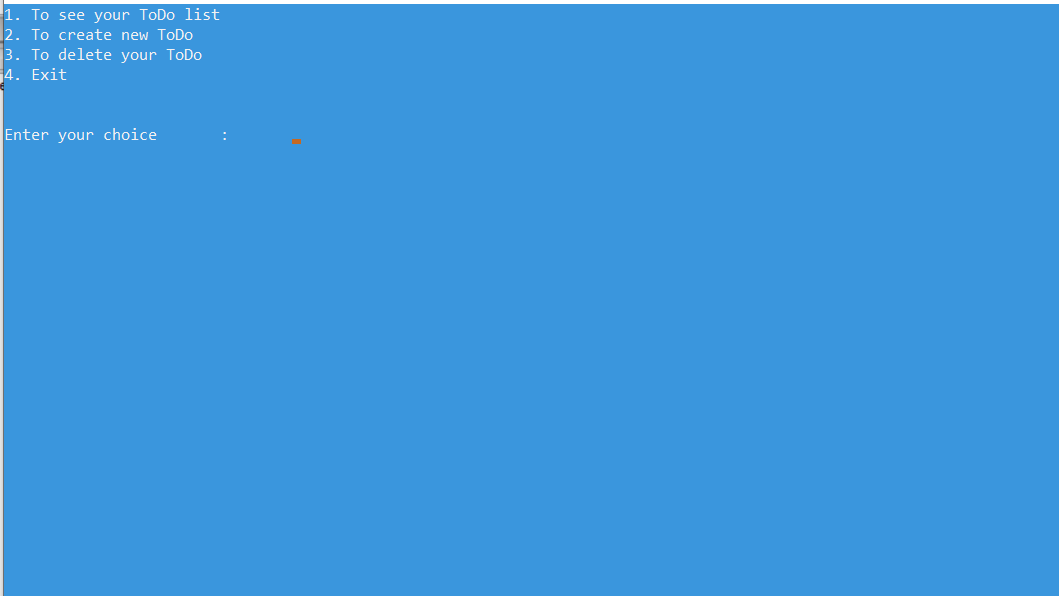• User Presses 2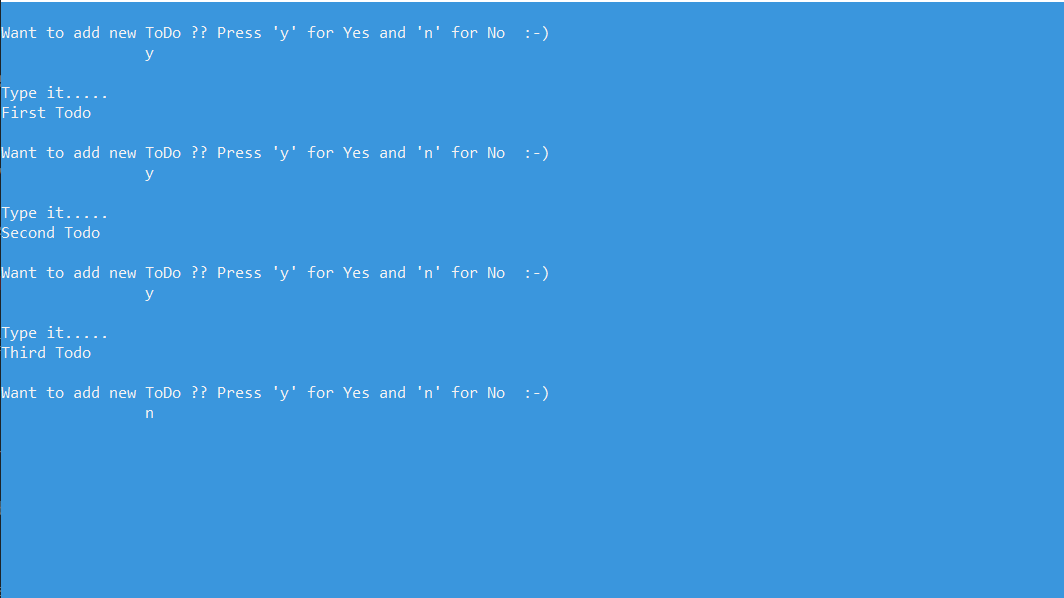• User Presses 1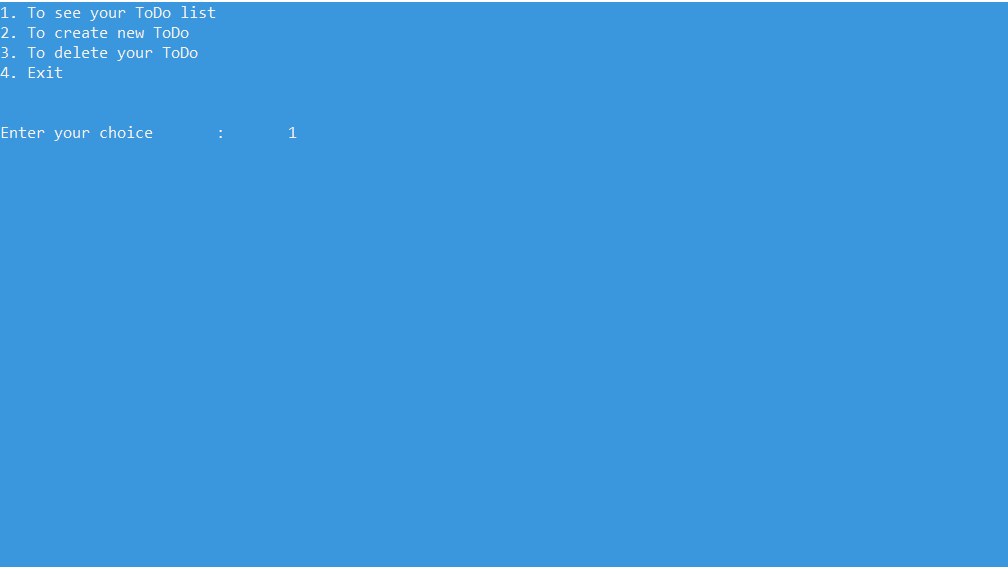• Displaying ToDos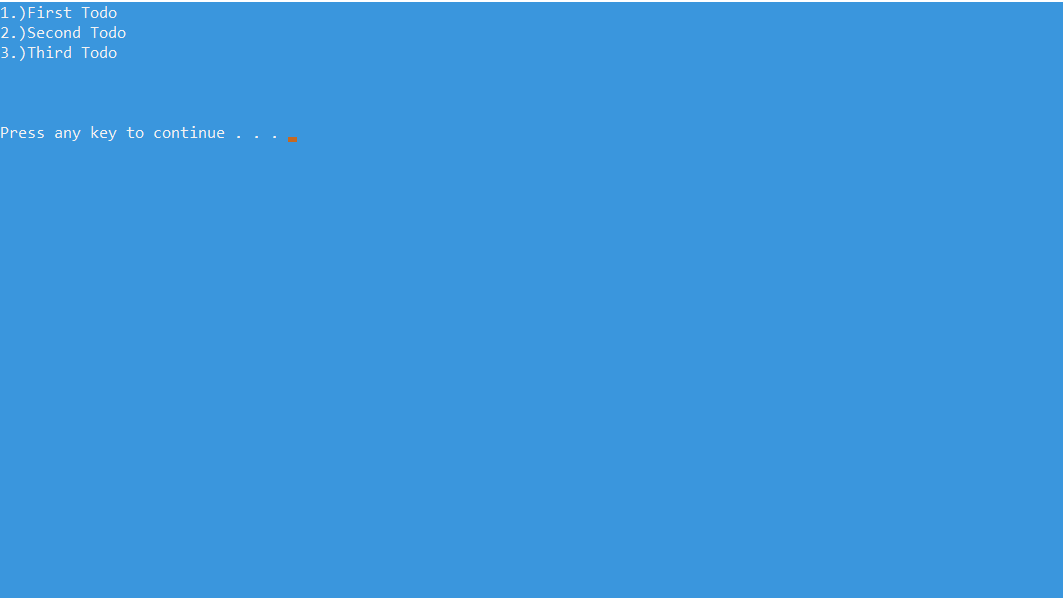• Deleting a ToDo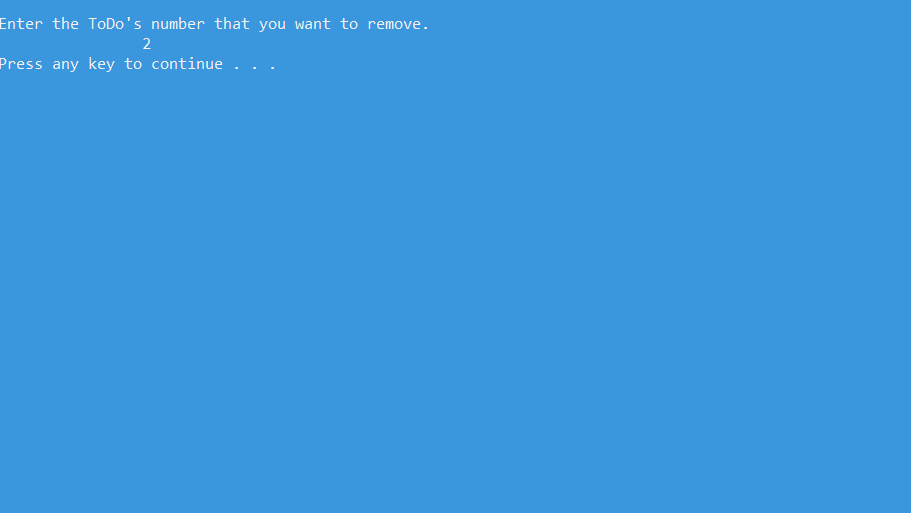• Displaying ToDos after Deleting a ToDo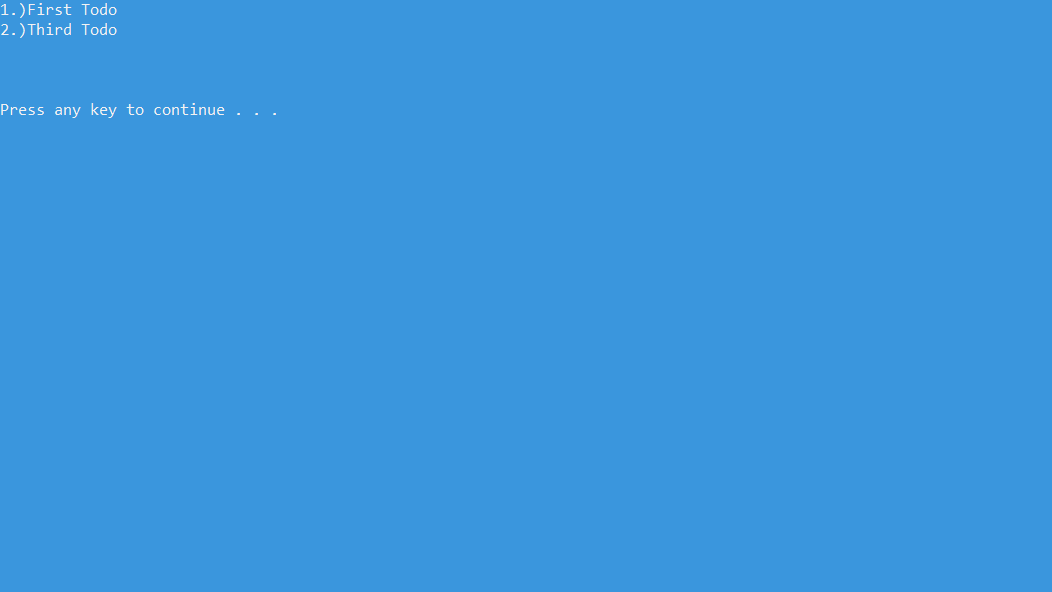• User Presses 4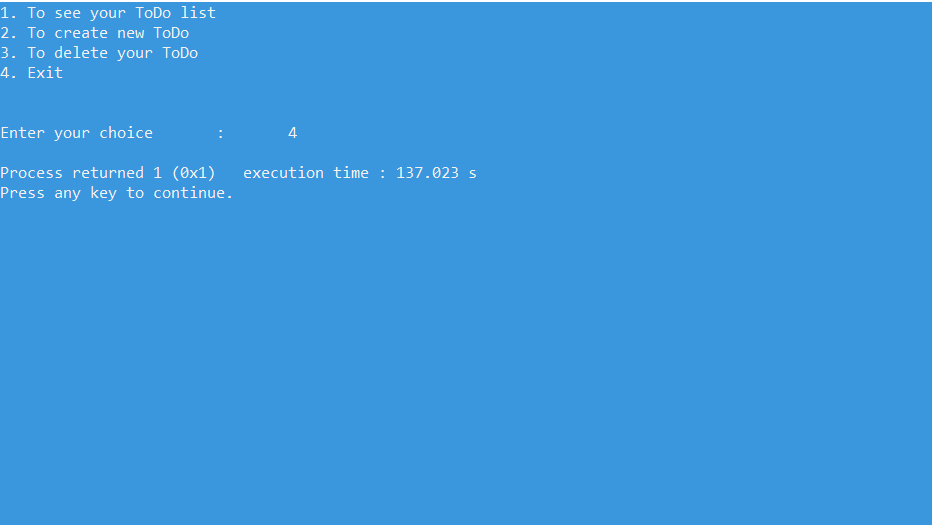Want to learn from the best curated videos and practice problems, check out the C++ Foundation Course for Basic to Advanced C++ and C++ STL Course for foundation plus STL.  To complete your preparation from learning a language to DS Algo and many more,  please refer Complete Interview Preparation Course.

My Personal Notes arrow_drop_up